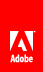# Node set functions

This section describes the functions that operate on schema nodes that are available in expressions.

## count

Returns the number of nodes in a given node set.

#### Syntax

`    count(node-set)`

#### Parameters

node-set is the path to a node in the process data model.

#### Returns

A number that holds the number of nodes.

## last

Returns a number equal to the context size from the expression evaluation context.

#### Syntax

`    last()`

None.

number.

## position

Returns a number equal to the context position from the expression evaluation context.

#### Syntax

`    position()`

None.

number.

## sum

Returns the sum, for each node in a given node-set, of the result of converting the string-values of the node to a number.

#### Syntax

`    sum(node-set)`

#### Parameters

node-set is a node in the XML.

#### Returns

number.// Ethnio survey code removed